The input signal given to a CE amplifier having a voltage gain of 150 is Vi=2cos(15t+π/3). The corresponding output signal will be

(a)300cos(15t+π/3)

(b)75cos(15t+2π/3)

(c)2cos(15t+5π/3)

(d)300cos(15t+4π/3)

High Yielding Test Series + Question Bank - NEET 2020

Difficulty Level:

The given graph represents V-I characteristic for a semiconductor device. Which of the following statement is correct?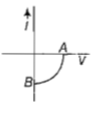(a) It is V-I characteristic for solar cell where point A represents open circuit voltage and point B short circuit current

(b) It is for a solar cell and points A and B represent open circuit voltage and current, respectively

(c) It is for a photodiode and points A and B represent open circuit voltage and current, respectively

(d) It is for a LED and points A and B represents open circuit voltage and short circuit current respectively

Concept Questions :-

Applications of PN junction
High Yielding Test Series + Question Bank - NEET 2020

Difficulty Level:

The barrier potential of a p-n junction depends on

(i)type of semiconductor material

(ii)amount of doping

(iii)temperature

Which one of the following is correct

1. (i) and (ii)only

2. (ii) only

3. (ii) and (iii)only

4. (i),(ii) and (iii)

Concept Questions :-

PN junction
High Yielding Test Series + Question Bank - NEET 2020

Difficulty Level:

In a n-type semiconductor, which of the following statement is true ?

(a) Electrons are majority carriers and trivalent atoms are dopants

(b) Electrons are minority carriers and pentavalent atoms are dopants

(c) Holes are minority carriers and pentavalent atoms are dopants

(d) Holes are majority carriers and trivalent atoms are dopants

Concept Questions :-

Types of semiconductors
High Yielding Test Series + Question Bank - NEET 2020

Difficulty Level:

The output (x) of logic circuit shown in figure will be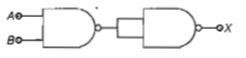(a) $X=\stackrel{=}{A}·\stackrel{=}{B}$

(b) $X=\overline{A.B}$

(c)$X=A·B$

(d)$X=\overline{A+B}$

Concept Questions :-

Logic gates
High Yielding Test Series + Question Bank - NEET 2020

Difficulty Level:

Two ideal diodes are connected to a battery as shown in the circuit. The current supplied by the battery is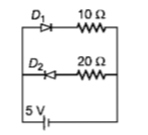(a)0.75A                              (b)zero

(c)0.25A                               (d)0.5A

Concept Questions :-

PN junction
High Yielding Test Series + Question Bank - NEET 2020

Difficulty Level:

In a CE transistor amplifier, the audio signal voltage across the collector resistance of 2  If the base resistance is 1 $k\Omega$ and the current amplification of the transistor is 100, the input signal voltage is

1. 0.1 V                                         2. 1.0 V

3. 1mV                                         4. 10mV

Concept Questions :-

Transistor
High Yielding Test Series + Question Bank - NEET 2020

Difficulty Level:

C and Si both have same lattice structure,having 4 bonding electrons in each.However, C is insulator whereas Si is intrinsic semiconductor. This is because

1. in case of C, the valence band is not completely filled at absolute zero temperature

2. in case of C,the condition band is partly filled even at absolute zero temperature

3. the four bonding electrons in the case of C lie in the second orbit,Whereas in the case of Si they lie in the third

4 .the four bonding electrons in the case of C lie in the third orbit, whereas for Si they lie in the fourth orbit

Concept Questions :-

Types of semiconductors
High Yielding Test Series + Question Bank - NEET 2020

Difficulty Level:

Transfer characteristic [output voltage $\left({V}_{0}\right)$ vs input voltage $\left({V}_{i}\right)$] for a base biased transistor in CE configuration is as shown in the figure.For using transistor as a switch, it is used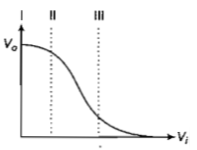(a) in region III

(b) both in region (I) and (III)

(c) in region II

(d) in region I

Concept Questions :-

Transistor
High Yielding Test Series + Question Bank - NEET 2020

Difficulty Level:

The figure shows a logic circuit with two inputs A and B and the output C.The voltage wave forms across A, B and C are as given.The logic circuit gate is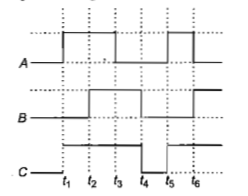1. OR gate                                          2. NOR gate

3. AND gate                                        4. NAND gate

Concept Questions :-

Logic gates